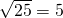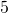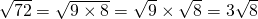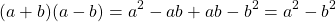# Surds

Any time we meet an irrational number in our work, we have the choice of decimalizing the number or leaving it in symbolic form.As can be seen from some of these ‘meanings’ the symbolic form captures a lot of information in a small amount of space on paper. The symbolic form is the exact value.

When we decimalize, we get an idea of the magnitude of the number. Eg,(2 d.p) This can be very useful especially if plotting the value on a graph. However the downside is that we lose accuracy. Rounded decimals are  not ‘exact answers’.

# Definition

A surd is the square root of a non-square number, or the cube root of a non-cube number etc. In other words, a radical whose value is not an integer.

The numbersandare not surds becauseand, which are both integers.

On the other hand the numbersandare surds asis not a perfect square andis not a perfect cube.

# Simplify a Surd (Square Root)

A surd (square root) can only be simplified if a perfect square is a factor of the radicand (the number under the square root).

The first 12 square numbers are:Example: SimplifyLet’s list the factors of 72.

The factors of 72 are:The factors that are also perfect squares are: 4, 9, 36 (also 1 but 1 is not useful here).

The highest is 36.

So,Suppose I selected 9 or 4 because I didn’t spot that 36 was a factor. In this case, I end up with another surd that can be simplified, so I just simplify again.

e.g.andTherefore,Simplify the square roots by writing as a product of a square number with a non square number:

# The Exponent Laws for Surds

As explained on the page Rational Exponents,.

The procedure to simplify surds works out because it follows the laws of exponents.In the case of square roots,Rewriting the example, we have# Rationalise a Denominator

A fraction that has a rational denominator is considered more simple than a fraction with an irrational denominator.

To rationalise a denominator, we multiply by the numberwhich retains the value of the fraction, however we choose the form ofto change the appearance of the fraction.

We use the fact thatforwith the exception of.

We also use the fact thatfor all## Example 1:

Rationalise the denominator of the fraction.

We use the facts:, and.The denominator, or the divisor, is now the integerwhich is a rational number.

In this following example we use the difference of two squares to eliminate the surds on the denominator. Using FOIL, we have.

## Example 2

Rationalise the denominator of the fraction.As this work is entirely numerical, we can check our answers on the calculator by evaluating the initial and final values to determine if they are indeed equal:# Practice

Try the Corbett Maths textbook questions on surds. Answers here. Worked solutions to select questions here: Solutions Corbett surds.